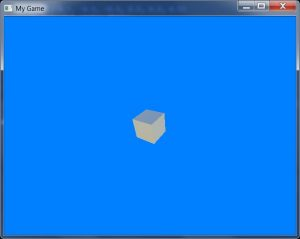## Getting started with mojo3d: Something to see!

Following on from the first part of this tutorial, where we created an empty scene (with nothing of interest to look at), we’ll now add a simple cube.```
#Import "<std>"
#Import "<mojo>"
#Import "<mojo3d>"

Using std..
Using mojo..
Using mojo3d..

Class MyGame Extends Window

Field scene:Scene
Field camera:Camera

Field cube:Model

Method New  (title:String = "My Game", width:Int = 640, height:Int = 480, flags:WindowFlags = WindowFlags.Resizable)
Super.New (title, width, height, flags)
End

Method OnCreateWindow () Override

scene						= New Scene
camera						= New Camera (Self)

Local cube_box:Boxf			= New Boxf (-0.5, -0.5, -0.5, 0.5, 0.5, 0.5)
Local cube_mat:PbrMaterial	= New PbrMaterial (Color.White)

cube						= Model.CreateBox (cube_box, 1, 1, 1, cube_mat)

End

Method OnRender (canvas:Canvas) Override

If Keyboard.KeyHit (Key.Escape) Then App.Terminate ()

RequestRender ()

scene.Update ()
camera.Render (canvas)

End

End

Function Main ()

New AppInstance
New MyGame

App.Run ()

End

```

This code adds a field, `cube:Model`, which we’ll use to store a Model-type entity (a 3D entity with a mesh and other related features).

The cube setup (in OnCreateWindow, after the scene and camera setup) consists of three lines:

1. `Local cube_box:Boxf = New Boxf (-0.5, -0.5, -0.5, 0.5, 0.5, 0.5)`

This creates a Box object (specifically of floating-point type, as denoted by the ‘f’), used to define a volume. The values define the far corners of the box — view these as two lots of three x, y, z values, each set of three defining an offset in space, so -0.5 in each direction, following by 0.5 in each direction, forming two corners into which the box will fit.

2. `Local cube_mat:PbrMaterial = New PbrMaterial (Color.White)`

This line creates a material that will be applied to the cube. (PBR refers to a form of realistic rendering that we won’t go into here.) The PbrMaterial New method can take a colour parameter, and we’re using one of mojo’s built-in Color-class definitions.

3. `cube = Model.CreateBox (cube_box, 1, 1, 1, cube_mat)`

And finally, the CreateBox helper method provided by the Model class takes the box we just set up, uses 1 for each of the x, y, z segment values (how many times the box is split along each axis) and the material to be applied.

Run the code and… nothing appears!

That’s because the camera and the cube occupy the same point in space; we can’t see anything because the inside faces of the cube (normally viewed from outside) are hidden by default.

Add another line after the CreateBox line:

```cube.Move (0, 0, 5)
```

This moves the cube 5 units along the z-axis, into the screen.

Run the code and the cube will appear.

Finally, for a bit of interest, add this line as the first line within OnRender:

```cube.Rotate (1, 2, 4)
```

Run again and the cube will rotate, 1 degree around the x-axis per update, 2 around the y-axis and 4 around the z.

Experiment with the code — change the material colour to another defined in the Color class (see docs, but examples include Color.Red, Color.Lime, etc); also, change the speed of rotation, or set two axes to 0 to see the effect.

You can also change the size of the box definition to scale the cube.

Try moving the camera backwards instead of moving the cube forwards — hint: both Camera and Model are derived from the Entity class, which supplies the Move method. You’ll need to replace the reference to the cube with the reference to the camera, and supply a negative z-value in place of the 5 in order to move the camera back. (If you already know how to check for keypresses, try moving the camera back and forth in OnRender!)

You can download the source code for this tutorial at GitHub. This post is covered by the example, “2. Hello, Stuff.monkey2”.